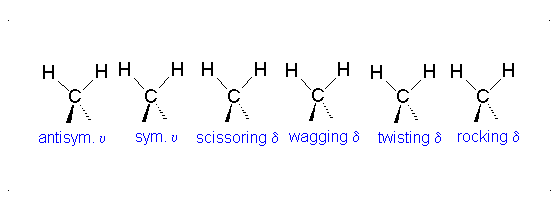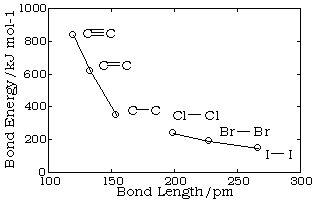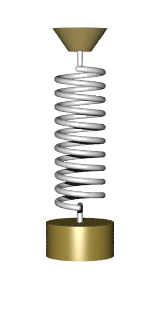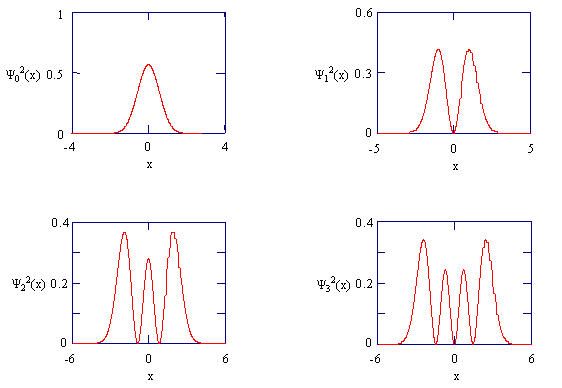Lecture 11: Vibrations

•• Contributed by Delmar Larsen
• Founder and Director at Libretexts

Last lecture focused on the extension of the 1D particle in a box to the 2-D and 3D cases. This is an easy problem since via a simple application of separation of variables (Worksheet 2) for each dimension (they act independently). From this we identified a few interesting phenomena including multiple quantum numbers ($$n_x$$, $$n_y$$ and $$n_z$$) and degeneracy (if the boxes are of the same length or multiples of each other) where multiple wavefunctions share the identical energy.

We were able to provide a quantitative backing in using the Heisenberg Uncertainty principle from wavefuctions, specifically in terms of the standard deviations of the probability distribution functions (i.e., wavefunction squared).

We ended the lecture on the five postulates of quantum mechanics, which ties together many aspects that we have been discussing.

Postulates of Quantum Mechanics in a Nutshell (you know all these already)

There are five assumptions made for the purpose of reasoning in quantum mechanics. These assumptions are called postulates, and they set the groundwork for quantum mechanics.

Postulate 1

The state of the system is completely specified by $$\psi$$. All possible information about the system can be found in the wavefunction $$\psi$$. For valid, well-behaved wavefunctions, normalized probability holds true, such that the integral over all space is equal to 1.

$\int_{-\infty}^\infty \psi^*(x)\psi(x)\;dx=1.$

What this means is that the chance to find a particle is 100% over all space. Wavefunctions must be
• single valued,
• continuous, and
• finite.
That means that the function must be a function, it cannot have an undefined slope, and the function cannot go to $$-\infty$$ or $$+\infty$$.

Postulate 2

For every observable in classical mechanics, there is a corresponding operator in quantum mechanics.
Name Observable Symbol Operator Symbol Operation
Table: Some common Operators in Quantum Mechanics
Position $$x$$ $$\hat{X}$$ Multiply by $$x$$
$$r$$ $$\hat{R}$$ Multiply by $$r$$
Momentum $$p_{x}$$ $$\hat{P_{x}}$$ -$$\imath \hbar \dfrac{d}{dx}$$
$$p$$ $$\hat{P}$$ -$$\imath$$$$\hbar$$[$$\text{i}$$$$\dfrac{d}{dx}$$+$$\text{j}$$$$\dfrac{d}{dy}$$+$$\text{k}$$$$\dfrac{d}{dz}$$]
Kinetic Energy $$K_{x}$$ $$\hat{K_{x}}$$ $$\dfrac{-(\hbar^{2})}{2m}$$$$\dfrac{d^{2}}{dx^{2}}$$
$$K$$ $$\hat{K}$$

$$\dfrac{-(\hbar^{2})}{2m}$$[$$\dfrac{d^{2}}{dx^{2}}$$+$$\dfrac{d^{2}}{dy^{2}}$$+$$\dfrac{d^{2}}{dz^{2}}$$]

Which can be simplified to
$$\dfrac{-(\hbar^{2})}{2m}$$$$\bigtriangledown^{2}$$

Potential Energy $$V(x)$$ $$\hat{V(x)}$$ Multiply by $$V(x)$$
$$V(x,y,z)$$ $$\hat{V(x,y,z)}$$ Multiply by $$V(x,y,z)$$
Total Energy $$E$$ $$\hat{E}$$ $$\dfrac{-(\hbar^{2})}{2m}$$$$\bigtriangledown^{2}$$ + $$V(x,y,z)$$
Angular Momentum $$L_{x}$$ $$\hat{L_{x}}$$ -$$\imath$$$$\hbar$$[$$\text{y}$$$$\dfrac{d}{dz}$$ - $$\text{z}$$$$\dfrac{d}{dy}$$]
$$L_{y}$$ $$\hat{L_{y}}$$ -$$\imath$$$$\hbar$$[$$\text{z}$$$$\dfrac{d}{dx}$$ - $$\text{x}$$$$\dfrac{d}{dz}$$]
$$L_{z}$$ $$\hat{L_{z}}$$ -$$\imath$$$$\hbar$$[$$\text{x}$$$$\dfrac{d}{dy}$$ - $$\text{y}$$$$\dfrac{d}{dx}$$]

Postulate 3

If $$\hat{A}$$$$\psi(x)$$ produces eigenvalues $$\{a\}$$, where

$\hat{A} \psi_n(x) = a_n \psi_n(x)$

then the set $$\{a_n\}$$ is the set of values (and only those values) that can be observed.

Postulate 4

For a normalized wavefunction, the average value of any observable with operation $$\hat{A}$$ is given by

$\langle a \rangle = \int_{-\infty}^\infty \psi^*(x) \hat{A}\ \psi(x) dx$

Postulate 5

Wavefunctions evolve in time, given by the Time-dependent Schrödinger Equation

$\hat{H}\Psi(x,t) = \imath \hbar \dfrac{d(\Psi(x,t))}{dt}$

where

$\Psi(x,t) = \psi(x)f(t)$

Using the Separation of Variables technique results in

$f(t)= e^\frac {-iEt}{\hbar} = e^{-it\omega}$

Commuting Operators

One important property of operators is that the order of operation matters. Thus:

$\hat{A}{\hat{E}f(x)} \not= \hat{E}{\hat{A}f(x)} \label{4.6.3}$

unless the two operators commute. Two operators commute if the following equation is true:

$\left[\hat{A},\hat{E}\right] = \hat{A}\hat{E} - \hat{E}\hat{A} = 0 \label{4.6.4}$

To determine whether two operators commute first operate $$\hat{A}\hat{E}$$ on a function $$f(x)$$. Then operate$$\hat{E}\hat{A}$$ the same function $$f(x)$$. If the same answer is obtained subtracting the two functions will equal zero and the two operators will commute.

Example $$\PageIndex{1}$$

Do $$\hat{A}$$ and $$\hat{E}$$ commute if

$\hat{A} = \dfrac{d}{dx}$

and

$\hat{E} = x^2$

Solution

This requires evaluating $$\left[\hat{A},\hat{E}\right]$$, which requires solving for $$\hat{A} \{\hat{E} f(x)\}$$ and $$\hat{E} \{\hat{A} f(x)\}$$ for arbitrary wavefunction $$f(x)$$ and asking if they are equal.

$\hat{A} \{\hat{E} f(x)\} = \hat{A}\{ x^2 f(x) \}= \dfrac{d}{dx} \{ x^2 f(x)\} = 2xf(x) + x^2 f'(x)$

From the product rule of differentiation.

$\hat{E} \{\hat{A}f(x)\} = \hat{E}\{f'(x)\} = x^2 f'(x)$

Now ask if they are equal

$\left[\hat{A},\hat{E}\right] = 2x f(x) + x^2 f'(x) - x^2f'(x) = 2x f(x) \not= 0$

Therefore the two operators do not commute.

Example $$\PageIndex{2}$$

Do $$\hat{B}$$ and $$\hat{C}$$ commute if

$\hat{B}= \dfrac {h} {x}$

and

$\hat{C}\{f(x)\} = f(x) +3$

Solution
This requires evaluating $$\left[\hat{B},\hat{C}\right]$$ like in Example $$\PageIndex{1}$$.

$\hat{B} \{\hat{C}f(x)\} = \hat{B}\{f(x) +3\} = \dfrac {h}{x} (f(x) +3) = \dfrac {h f(x)}{x} + \dfrac{3h}{x}$

$\hat{C} \{\hat{B}f(x)\} = \hat{C} \{ \dfrac {h} {x} f(x)\} = \dfrac {h f(x)} {x} +3$

Now ask if they are equal

$$\left[\hat{B},\hat{C}\right] = \dfrac {h f(x)} {x} + \dfrac {3h} {x} - \dfrac {h f(x)} {x} -3 \not= 0$$

The two operators do not commute.

Heisenberg Uncertainty Principle Redux (Redux Redux)

Although it will not be proven here, there is a general statement of the uncertainty principle in terms of the commutation property of operators. If two operators $$\hat {A}$$ and $$\hat {B}$$ do not commute, then the uncertainties (standard deviations σ) in the physical quantities associated with these operators must satisfy

$\sigma _A \sigma _B \ge \left| \int \psi ^* [ \hat {A} \hat {B} - \hat {B} \hat {A} ] \psi d\tau \right| \label{4-52}$

where the integral inside the square brackets is called the commutator, and ││signifies the modulus or absolute value. If $$\hat {A}$$ and $$\hat {B}$$ commute, then the right-hand-side of equation $$\ref{4-52}$$ is zero, so either or both $$σ_A$$ and $$σ_B$$ could be zero, and there is no restriction on the uncertainties in the measurements of the eigenvalues $$a$$ and $$b$$.

Vibrations

The motion of two particles in space can be separated into translational, vibrational, and rotational motions.

Different ways of visualizing the 6 degrees of freedom of a diatomic molecule. (CM: center of mass of the system, T: translational motion, R: rotational motion, V: vibrational motion.) Image used with permission (Public domain; Mythealias)

IR spectroscopy which has become so useful in identification, estimation, and structure determination of compounds draws its strength from being able to identify the various vibrational modes of a molecule. A complete description of these vibrational normal modes, their properties and their relationship with the molecular structure is the subject of this article.

Degree of freedom is the number of variables required to describe the motion of a particle completely. For an atom moving in 3-dimensional space, three coordinates are adequate so its degree of freedom is three. Its motion is purely translational. If we have a molecule made of N atoms (or ions), the degree of freedom becomes 3N, because each atom has 3 degrees of freedom. Furthermore, since these atoms are bonded together, all motions are not translational; some become rotational, some others vibration.

The degrees of vibrational modes for linear molecules can be calculated using the formula:

$3N-5 \label{1}$

The degrees of freedom for nonlinear molecules can be calculated using the formula:

$3N-6 \label{2}$

$$n$$ is equal to the number of atoms within the molecule of interest. The following procedure should be followed when trying to calculate the number of vibrational modes:

1. Determine if the molecule is linear or nonlinear (i.e. Draw out molecule using VSEPR). If linear, use Equation \ref{1}. If nonlinear, use Equation \ref{2}
2. Calculate how many atoms are in your molecule. This is your $$N$$ value.
3. Plug in your $$N$$ value and solve.
Atoms (very symmetric) Linear molecules (less symmetric) Non-linear molecules (most unsymmetric)
Translation (x, y, and z) 3 3 3
Rotation (x, y, and z) 0 2 3
Vibrations 0 3N − 5 3N − 6
Total (including Vibration) 3 3N 3N

Example 1: Water• The Symmetric Stretch (Example shown is an H2O molecule at 3685 cm-1)
• The Asymmetric Stretch (Example shown is an H2O molecule at 3506 cm-1)
• Bend (Example shown is an H2O molecule at 1885 cm-1)

A linear molecule will have another bend in a different plane that is degenerate or has the same energy. This accounts for the extra vibrational mode.

Example 2: Carbon DioxideExample 3: The Methylene GroupIt is important to note that there are many different kinds of bends, but due to the limits of a 2-dimensional surface it is not possible to show the other ones.

The frequency of these vibrations depend on the inter atomic binding energy which determines the force needed to stretch or compress a bond.

Properties of a Molecular Bond

What do we know about bonds from general chemistry?

1. Breaking a bond always requires energy and hence making bonds always release energy.
2. Bond length
3. Bond energy (or enthalpy or strength)

The potential energy of a system of two atoms depends on the distance between them. At large distances the energy is zero, meaning “no interaction”. At distances of several atomic diameters attractive forces dominate, whereas at very close approaches the force is repulsive, causing the energy to rise. The attractive and repulsive effects are balanced at the minimum point in the curve.The internuclear distance at which the potential energy minimum occurs defines the bond length. This is more correctly known as the equilibrium bond length, because the two atoms will always vibrate about this distance.

Bond lengths depend mainly on the sizes of the atoms, and secondarily on the bond strengths, the stronger bonds tending to be shorter. Bonds involving hydrogen can be quite short; The shortest bond of all, H–H, is only 74 pm. Multiply-bonded atoms are closer together than singly-bonded ones; this is a major criterion for experimentally determining the multiplicity of a bond. This trend is clearly evident in the above plot which depicts the sequence of carbon-carbon single, double, and triple bonds.In general, the stronger the bond, the smaller will be the bond length.

Reduced mass (Converting two atoms moving into one)

The internal motions of vibration and rotation for a two-particle system can be described by a single reduced particle with a reduced mass $$μ$$ located at $$r$$. For a diatomic molecule, In the below figure, the vector $$\vec{r}$$ corresponds to the internuclear axis. The magnitude or length of $$r$$ is the bond length, and the orientation of $$r$$ in space gives the orientation of the internuclear axis in space. Changes in the orientation correspond to rotation of the molecule, and changes in the length correspond to vibration. The change in the bond length from the equilibrium bond length is the vibrational coordinate for a diatomic molecule.The diagram shows the coordinate system for a reduced particle. $$R_1$$ and $$R_2$$ are vectors to $$m_1$$ and $$m_2$$. $$R$$ is the resultant and points to the center of mass. (b) Shows the center of mass as the origin of the coordinate system, and (c) expressed as a reduced particle.

The Classical Harmonic Oscillator

Simple harmonic oscillators about a potential energy minimum can be thought of as a ball rolling frictionlessly in a dish (left) or a pendulum swinging frictionlessly back and forth. The restoring forces are precisely the same in either horizontal direction.Simple image of a ball oscillating in a potential. Image used with permission from Wikipedia.

Recall that the Hamiltonian operator $$\hat{H}$$ is the summation of the kinetic and potential energy in a system. There are several ways to approximate the potential function $$V$$, but the two main means of approximation are done by using a Taylor series expansion, and the Morse Potential. The vibration of a diatomic is akin to an oscillating mass on a spring.An undamped spring–mass system undergoes simple harmonic motion. Image used with permission from Wikipedia.

The classical forces in chemical bonds can be described to a good approximation as spring-like or Hooke's law type forces. This is true provided the energy is not too high. Of course, at very high energy, the bond reaches its dissociation limit, and the forces deviate considerably from Hooke's law. It is for this reason that it is useful to consider the quantum mechanics of a harmonic oscillator.

We will start in one dimension. Note that this is a gross simplification of a real chemical bond, which exists in three dimensions, but some important insights can be gained from the one-dimensional case. The Hooke's law force is

$F(x)=-k(x-x_0)$

where $$k$$ is the spring constant. This force is derived from a potential energy

$V(x)=\dfrac{1}{2}k(x-x_0)^2$

Let us define the origin of coordinates such that $$x_0 =0$$. Then the potential energy

$\color{red} V(x)=\dfrac{1}{2}kx^2$

If a particle of mass $$m$$ is subject to the Hooke's law force, then its classical energy is

$\dfrac{p^2}{2m}+\dfrac{1}{2}kx^2 =E$

Thus, we can set up the Schrödinger equation:

$\left [ -\dfrac{(\hbar)^2}{2m}\dfrac{d^2}{dx^2}+\dfrac{1}{2}kx^2 \right ]\psi (x)=E\psi (x)$

In this case, the Hamiltonian is

$\hat{H}=-\dfrac{(\hbar)^2}{2m}\dfrac{d}{dx^2}+\dfrac{1}{2}kx^2$

Since $$x$$ now ranges over the entire real line $$x\epsilon (-\infty ,\infty)$$, the boundary conditions on $$\psi (x)$$ are conditions at $$x=\pm \infty$$. At $$x= \pm \infty$$, the potential energy becomes infinite. Therefore, it must follow that as $$x \rightarrow \pm \infty$$, $$\psi (x)\rightarrow 0$$. Hence, we can state the boundary conditions as $$\psi (\pm \infty)=0$$.

Solving this differential equation is not an easy task, so we will not attempt to do it. Here, we simply quote the allowed energies and some of the wave functions. The allowed energies are characterized by a single integer $$v$$, which can be $$0,1,2,...$$ and take the form

$\color{red} E_v =\left ( v+\dfrac{1}{2} \right )h\nu_1 \label{BigEq}$

where $$\nu$$ is the frequency of the oscillation (of a single mass on a spring):

$\nu_{1} =\dfrac{1}{2\pi}\sqrt{\dfrac{k}{m}}$

$$\nu_1$$ is the fundamental frequency of the mechanical oscillator which depends on the force constant of the spring and a single mass of the attached (single) body and independent of energy imparted on the system. when there are two masses involved in the system (e.g., a vibrating diatomic), then the mass used in Equation $$\ref{BigEq}$$ becomes is a reduced mass:

$\color{red} \mu = \dfrac{m_1 m_2}{m_1+m_2} \label{14}$

The fundamental vibrational frequency is then rewritten as

$\nu = \dfrac{1}{2\pi} \sqrt{\dfrac{k}{\mu}} \label{15}$

Do not confuse $$v$$ the quantum number for harmonic oscillator with $$\nu$$ the fundamental frequency of the vibration

The natural frequency $$\nu$$ can be converted to angular frequency $$\omega$$ via

$\omega = 2\pi \nu$

Then the energies in Equation $$\ref{BigEq}$$ can be rewritten in terms of the fundamental angular frequency as

$\color{red} E_v =\left ( v+\dfrac{1}{2} \right )\hbar \omega \label{BigEq2}$

Now the eigenstates

Now we can define the parameter (for convenience)

$\alpha =\dfrac{\sqrt{km}}{\hbar}=\dfrac{m\omega}{\hbar}=\dfrac{4\pi ^2m\nu}{h}$

the first few wave functions are

\begin{align*}\psi_0 (x) &= \left ( \dfrac{\alpha}{\pi} \right )^{1/4}e^{-\alpha x^2 /2}\\ \psi_1(x) &= \left ( \dfrac{4\alpha ^3}{\pi} \right )^{1/4}xe^{-\alpha x^2 /2}\\ \psi_2 (x) &= \left ( \dfrac{\alpha}{4\pi} \right )^{1/4}(2\alpha x^2 -1)e^{-\alpha x^2/2}\\ \psi_3 (x) &= \left ( \dfrac{\alpha ^3}{9\pi} \right )^{1/4}(2\alpha x^3 -3x)e^{- \alpha x^2 /2}\end{align*}

You should verify that these are in fact solutions of the Schrödinger equation by substituting them back into the equation with their corresponding energies. The figure below shows these wave functionsThe harmonic oscillator wavefunctions describing the four lowest energy states.

The figure below shows these wave functions and the corresponding probability densities: $$p_n (x)=\psi_{n}^{2}(x)$$:The probability densities for the four lowest energy states of the harmonic oscillator.

Note that in contrast to a particle in an infinite high box, $$x\epsilon (-\infty ,\infty)$$, so the normalization conditionfor each eignestate is

$\int_{-\infty}^{\infty}\psi_{n}^{2}(x)dx=1$

Despite this, because the potential energy rises very steeply, the wave functions decay very rapidly as $$|x|$$ increases from 0 unless $$n$$ is very large. This is discussed as tunneling elsewhere.

Another way to show solutions to harmonic oscillators. (left) Wavefunction representations for the first eight bound eigenstates, n = 0 to 7. The horizontal axis shows the position x. Note: The graphs are not normalized, and the signs of some of the functions differ from those given in the text. (right) Corresponding probability densities.Images used with permission (CC BY-SA 3.0; AllenMcC.)